• 当前位置:我的异常网» C语言»创建二叉树是如何输入创建二叉树是如何输入？www.myexceptions.net网友分享于：2013-06-14浏览：51次创建二叉树是怎么输入？？？？？？？？？？？#include#include#define NULL 0#...

当前位置:我的异常网» C语言 » 创建二叉树是如何输入？

创建二叉树是如何输入？

www.myexceptions.net  网友分享于：2013-06-14  浏览：51次

创建二叉树是怎么输入？？？？？？？？？？？

#include

#include

#define NULL 0

#define LEN_T sizeof(BTNode)

#define LEN_Q sizeof(QNode)

#define LEN_S 100

typedef char ElemType;

typedef struct BTNode

{

ElemType data;

struct BTNode *lchild,*rchild;

}BTNode,*BTree;

typedef struct QNode

{

BTree data;

struct QNode *next;

}QNode,*Queue;

typedef struct StackElemType

{

BTree data;

int f;//f=0：遍历左子树 f=1：遍历右子树

}StackElemType;

void CreateTree(BTree *T)

{

char c;

c=getchar();

if (c=='#')

(*T)=NULL;

else

{

(*T)=(BTree)malloc(LEN_T);

CreateTree(&(*T)->lchild);

(*T)->data=c;

CreateTree(&(*T)->rchild);

}

}

void Xian(BTree T)

{

if(T)

{

printf("%2c",T->data);

Xian(T->lchild);

Xian(T->rchild);

}

}

void D_Xian(BTree T)

{

BTree p=T,Stack[LEN_S];

int top=0;

do{

while(p)

{

printf("%2c",p->data);

Stack[top++]=p;

p=p->lchild;

}

if(top>0)

{

p=Stack[--top];

p=p->rchild;

}

}while(top>0||p!=NULL);

}

void Zhong(BTree T)

{

if(T)

{

Zhong(T->lchild);

printf("%2c",T->data);

Zhong(T->rchild);

}

}

void D_Zhong(BTree T)

{

BTree p=T,Stack[LEN_S];

int top=0;

do{

while(p)

{

Stack[top++]=p;

p=p->lchild;

}

if(top>0)

{

p=Stack[--top];

printf("%2c",p->data);

p=p->rchild;

}

}while(top>0 || p);

}

void Hou(BTree T)

{

if(T)

{

Hou(T->lchild);

Hou(T->rchild);

printf("%2c",T->data);

}

}

void D_Hou(BTree T)

{

StackElemType Stack[LEN_S];

BTree p=T;

int top=0;

do{

while(p)

{

Stack[top].f=0;

Stack[top].data=p;

p=p->lchild;

top++;

}

if(top>0)

{

while(Stack[top-1].f==1)

{

p=Stack[--top].data;

printf("%2c",p->data);

}

if(top>0)

{

Stack[top-1].f=1;

p=Stack[top-1].data;

p=p->rchild;

}

}

}while(top>0);

}

//队列开始

void InitQueue(Queue *front,Queue *rear)

{

(*front)=(*rear)=(Queue)malloc(LEN_Q);

}

void EnQueue(Queue *rear,BTree p)

{

Queue q;

q=(Queue)malloc(LEN_Q);

q->data=p;

q->next=NULL;

(*rear)->next=q;

(*rear)=q;

}

文章评论

展开全文• #include #include #include using namespace std;... } 这是演示示例的逻辑结构图，本例中以编译器输入#为结束符号，即表示指向空 所以在编译器中的输入应为： ABC##DE###FG##H#I## 下图是对应代码的运行结果
#include<iostream>
#include<time.h>
#include<Windows.h>
using namespace std;
#define SIZE 100
#pragma warning(disable:4996)
typedef struct node
{
char data;
struct node* lchild;
struct node* rchild;

}*TNode,Tnode;
TNode createtree();//建立二叉树思路一
void Createtree(TNode &Tree/* 也可以传参为TNode *Tree或Tnode **Tree,不用这两种是引用可以减轻代码出错率 */);//建立二叉树思路二
/*二叉树整体建立思想就是利用递归进行元素存放*/
/*关于建立二叉树传参的一些解释说明
此处的TNode &Tree是直接对Tree指针进行引用，所以在给指针分配空间时不需要用(*Tree)->lchild;而是直接用Tree->lchild,当然这也体现
了方法一的简洁性，即直接放弃通过函数外传参函数内初始化参数而是直接将所有操作在函数内完成，并返回需要的数据。
*/
void Aprinttree(TNode T);//先序遍历输出二叉树
void Bprinttree(TNode T);//中序遍历输出二叉树
void Cprinttree(TNode T);//后序遍历输出二叉树
void floortree(TNode T);//层序遍历输出二叉树
void destroytree(TNode T);//销毁二叉树
int gettreeheight(TNode T);//获取二叉树的高度
void Sumnode(TNode T, int &sum);//获取二叉树的结点总数
int zeronodecount(TNode T, int &sum);//求叶子结点个数
int onenodecount(TNode T, int &sum);//求度为1的结点个数
int main()
{
int sumnode=0;
int zeronode = 0;
int onenode = 0;
int high;
TNode T;
cout << "请以先序遍历录入二叉树：";
Createtree(T);//T = createtree()或Createtree(&T);
cout << "先序遍历打印此二叉树结果为：";
Aprinttree(T); cout << endl;
cout << "中序遍历打印此二叉树结果为：";
Bprinttree(T); cout << endl;
cout << "后序遍历打印此二叉树结果为：";
Cprinttree(T); cout << endl;
cout << "层序遍历打印此二叉树结果为：";
floortree(T); cout << endl;
high = gettreeheight(T);
cout << "此二叉树的高度为：" << high << endl;
Sumnode(T, sumnode);
cout << "此二叉树的结点个数为：" << sumnode << endl;
zeronodecount(T, zeronode);
cout << "此二叉树的叶子结点个数为：" << zeronode << endl;
onenodecount(T, onenode);
cout << "此二叉树的度为1的结点个数为：" << onenode << endl;
cout << "此二叉树的度为2的结点个数为：" << zeronode-1 << endl;
destroytree(T);
return 0;
}
TNode createtree()//建立二叉树方法一
{
Tnode *Tree;
char ch;
cin >> ch;
if (ch == '#')Tree = NULL;
else
{
Tree = new Tnode;
Tree->data = ch;
Tree->lchild = createtree();
Tree->rchild = createtree();
}
return Tree;
}
void Createtree(TNode &Tree/* 也可以传参为TNode *Tree或Tnode **Tree,不用这两种是引用可以减轻代码出错率*/)//建立二叉树方法二
{
char ch;
cin >> ch;
if (ch == '#')Tree = NULL;
else
{
Tree = new Tnode;
Tree->data = ch;
Createtree(Tree->lchild);
Createtree(Tree->rchild);
}
/*这是传入参数为TNode *Tree或Tnode **Tree时对应的代码
char ch;
cin >> ch;
if (ch == '#')*Tree = NULL;
else
{
*Tree = new Tnode;
(*Tree)->data = ch;
Createtree(&(*Tree)->lchild);
Createtree(&(*Tree)->rchild);
}
*/
}
void Aprinttree(TNode T)//先序遍历输出二叉树
{
if(T)
{
cout << T->data;
Aprinttree(T->lchild);
Aprinttree(T->rchild);
}
}
void Bprinttree(TNode T)//中序遍历输出二叉树
{
if (T)
{
Bprinttree(T->lchild);
cout << T->data;
Bprinttree(T->rchild);
}
}
void Cprinttree(TNode T)//后序遍历输出二叉树
{
if (T)
{
Cprinttree(T->lchild);
Cprinttree(T->rchild);
cout << T->data;
}
}
void floortree(TNode T)//层序遍历输出二叉树
{
TNode temp;
int in = 0, out = 0;
temp[in++] = T;
while (in > out)
{
if (temp[out])
{
cout << temp[out]->data;
temp[in++] = temp[out]->lchild;
temp[in++] = temp[out]->rchild;
}
out++;
}

}
void destroytree(TNode T)//销毁二叉树
{
if (T != NULL)
{
destroytree(T->lchild);
destroytree(T->rchild);
delete T;
}
}
int gettreeheight(TNode T)//获取二叉树的高度
{
if (T == NULL)return 0;
else
{
int m = gettreeheight(T->lchild);
int n = gettreeheight(T->rchild);
return m >= n ? m + 1 : n + 1;
}
}
void Sumnode(TNode T,int &sum)//获取二叉树的结点总数
{
if (T == NULL)return ;
else
{
Sumnode(T->lchild,sum);
Sumnode(T->rchild, sum);
sum ++;
}
}
int zeronodecount(TNode T,int &sum)//求叶子结点个数
{
if (T == NULL)return 0;
int m = zeronodecount(T->lchild,sum);
int n = zeronodecount(T->rchild,sum);
if (m == 0 && n == 0)
{
sum++;
return 1;
}
else return 1;
}
int onenodecount(TNode T, int &sum)//求度为1的结点个数
{
if (T == NULL)return 0;
int m = onenodecount(T->lchild, sum);
int n = onenodecount(T->rchild, sum);
if (m != n)
{
sum++;
return 1;
}
else return 1;
}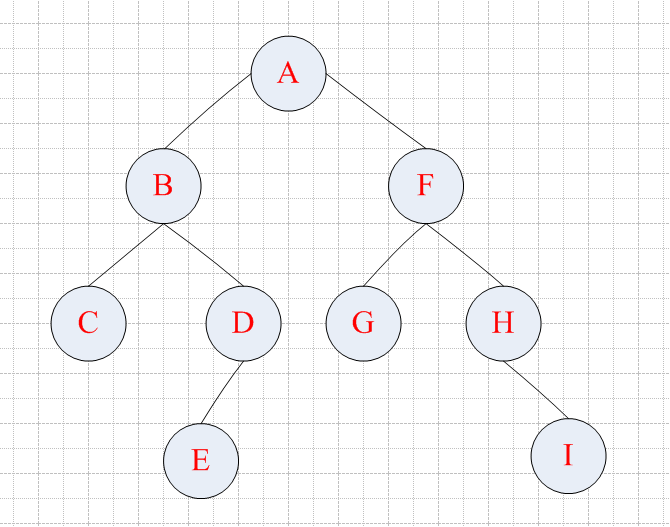这是演示示例的逻辑结构图，本例中以编译器输入#为结束符号，即表示指向空
所以在编译器中的输入应为： ABC##DE###FG##H#I##
下图是对应代码的运行结果展开全文数据结构 二叉树 算法
• 二叉树创建 三.二叉树的遍历 1.先序遍历 2.中序遍历 3.后序遍历 4.层序遍历 一.二叉树的存储结构 二叉树的基本存储结构如下所示 leftchild:存储左孩子节点的地址 rightchild:存储右孩子节点的地址 ...

目录

一.二叉树的存储结构

二.二叉树的创建

三.二叉树的遍历

1.先序遍历

2.中序遍历

3.后序遍历

4.层序遍历

一.二叉树的存储结构

二叉树的基本存储结构如下所示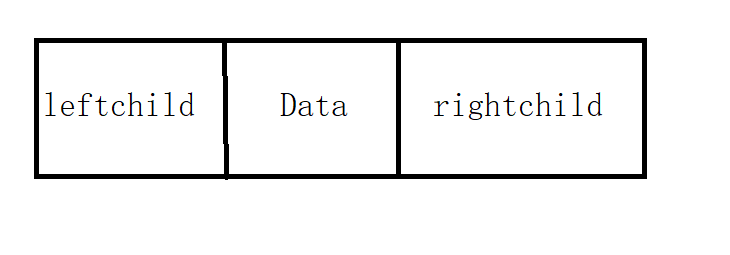leftchild:存储左孩子节点的地址

rightchild:存储右孩子节点的地址

data：当前节点存储的信息

/*节点结构*/
typedef struct node
{
char a;
struct node* leftchild, * rightchild;
}Bitree;

二.二叉树的创建

创建一棵二叉树的关键在于如何将一个一个的节点按照树的脉络连接起来，在这里我们选择用一棵最小的满二叉树来说明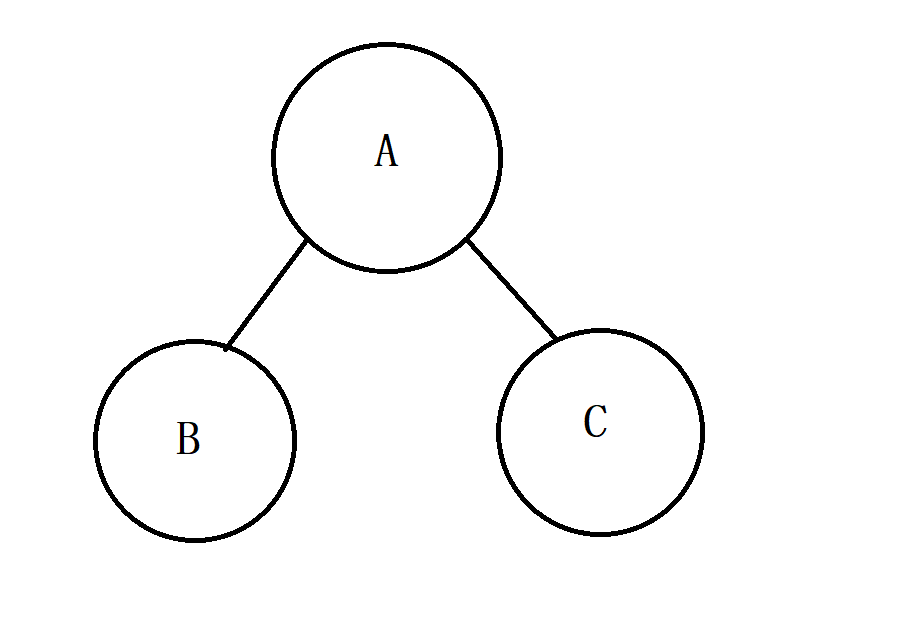我们需要首先创建根节点，并且向里面保存数据，然后接着在向左孩子里面输入数据，最后是右孩子，其实这种从根节点开始，依次访问左节点，右节点的方式叫做先序（先序是针对根节点的操作来说的）

对于一个最小的满二叉树我们的做法是这样，那对于下面二叉树我们的做法是什么呢？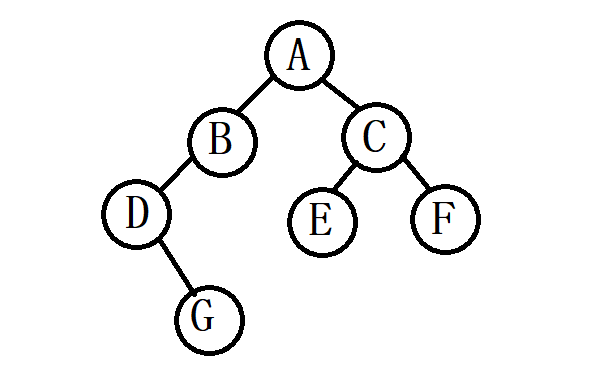创建这样的一个二叉树需要什么步骤呢？ 首先创建A节点，然后是B,C节点，在B节点的下方只有一个节点所以我们在创建一个D节点？如果这样做的话，我们就不知道在某一个节点的下面是否存在一个节点或者是两个节点抑或是没有节点，所以对于这样的问题，我们所采取的措施，是对于每一个非叶节点，如果没有两个节点，则零一节点置空，那么对于节点来说，就都是两个节点，新的问题出现了，那怎么去置空呢？  那我们就在输入数据的时候对于没有数据的节点，输入‘#’，如果等于字符等于‘#’，就返回NULL。

在这里我们依然采用递归的算法

void create(Bitree** T)
{
char a;
scanf_s("%c", &a);
if (a == '#')
(*T) = NULL;
else
{
(*T) = (Bitree*)malloc(sizeof(Bitree));
(*T)->a = a;
create(&(*T)->leftchild);
create(&(*T)->rightchild);
}
}

三.二叉树的遍历

1.先序遍历

先序遍历中的先序依然是对于树的根节点的操作顺序，其顺序为

1.访问根节点

2.前序遍历根节点的左子树

3.前序遍历根节点右子树

void xian(Bitree* T)
{
if (T)
{
printf("%c", T->a);
xian(T->leftchild);
xian(T->rightchild);
}
}

2.中序遍历

1.前序遍历根节点的左子树

2.访问根节点

3.前序遍历根节点右子树

void zhong(Bitree* T)
{
if (T)
{
zhong(T->leftchild);
printf("%c", T->a);
zhong(T->rightchild);
}
}

3.后序遍历

1.后序遍历根节点的左子树

2.后序遍历根节点右子树

3.访问根节点

void hou(Bitree* T)
{
if (T)
{
hou(T->leftchild);
hou(T->rightchild);
printf("%c", T->a);
}
}

4.层序遍历

层序遍历是将一个二叉树从上到下，从左到右一层一层的把所有的数据都显示出来，在所有未被访问的节点的集合中，排列在已访问节点集合中最前面的节点的左子树最先被访问，然后是该节点的的右子树的根节点，如果将所有已被访问的节点放在一个队列中，那么所有未被访问节点的次序姐可以由存在队列中一访问节点的出对次序决定

其算法为：

1.初始化一个队列

2.将根节点指针入队列

3.当队列非空时，循环执行a-c的步骤

a.出队列取得一个节点指针，访问该节点

b.若该节点的左子树非空，则将该结点的左孩子指针入队列

c.若该节点的右子树非空，就将该节点的右孩子指针入队列

4.结束

具体代码如下

队列的子函数

void initiate(Q** O)
{
(*O) = (Q*)malloc(sizeof(Q));
(*O)->front = NULL;
(*O)->last = NULL;
}

/*入队列*/
void append(Q* O, Bitree* p)
{
Node* q;
q = (Node*)malloc(sizeof(Node));
q->p = p;
q->next = NULL;
if (O->last != NULL)O->last->next = q;
O->last = q;
if (O->front == NULL)O->front = q;
}

void out(Q* O, Bitree** p)
{
Node* q;
if (O->front == NULL)
{
printf("当前队列无元素！\n");
return;
}
else
{
(*p) = O->front->p;
q = O->front;
O->front = O->front->next;
if (O->front == NULL)O->last = NULL;
free(q);
return;
}
}

层序遍历函数

/*层序遍历*/
void ceng(Bitree* T)
{
Q* O;
Bitree* p;
initiate(&O);
append(O, T);
while (O->last)
{
out(O, &p);
printf("%c", p->a);
if (p->leftchild)append(O, p->leftchild);
if (p->rightchild)append(O, p->rightchild);
}
printf("\n");
}

函数测试如下所示展开全文1024程序员节 数据结构 c语言
• //再来递归两下 } 扩展资料 树的定义还需要强调以下两点： 1）n>0时根结点是唯一的，不可能存在多个根结点，数据结构中的树只能有一个根结点。 2）m>0时，子树的个数没有限制，但它们一定是互不相交的。 由树的定义...

展开全部

代码如下：

char a；

int len，i；//i逐渐增加

void build(int s){

if(i==len) return；//已经建完树了

i++；

if(!tree[s].l) tree[s].l=c；//如果树的左边是空的，就给左边赋值

else tree[s].r=c；//反之

if(c!=' ') build(c)；

if(c!=' ') build(c)；//再来递归两下

}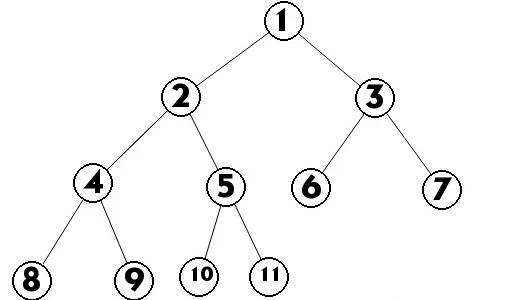扩展资料

树的定义还需要强调以下两点：

1)n>0时根结点是唯一的，不可能存在多个根结点，数据结构中的树只能有一个根结点。

2)m>0时，子树的个数没有限制，但它们一定是互不相交的。

由树的定义可以看出，树的定义使用了递归的方式。递归在树的学习过程中起着重要作用。结点拥有的子树数目称为结点的度。结点子树的根结点为该结点的孩子结点。相应该结点称为孩子结点的双亲结点。从根开始定义起，根为第一层，根的孩子为第二层，以此类推。树中结点的最大层次数称为树的深度或高度。

二叉树是n(n>=0)个结点的有限集合，该集合或者为空集(称为空二叉树)，或者由一个根结点和两棵互不相交的、分别称为根结点的左子树和右子树组成。

由二叉树定义以及图示分析得出二叉树有以下特点：

1)每个结点最多有两颗子树，所以二叉树中不存在度大于2的结点

2)左子树和右子树是有顺序的，次序不能任意颠倒。

3)即使树中某结点只有一棵子树，也要区分它是左子树还是右子树。

参考资料来源：

展开全文• 数据结构】（十一）二叉树创建（懒猫老师代码） （一）说明： 创建二叉树还是需要用到二叉树的遍历： 为什么要有返回值，是因为：返回值是数节点与节点相连的的桥梁，如果不返回则，建立的结点之间不相连，...线索二叉树 二叉树的创建和遍历 线索二叉树的存储结构 数据结构 算法
• 一、引言：大三上学期学了数据结构后就没再接触过数据结构的内容了，至今已经整整两年半了，已经把链表，队列，堆栈，树，二叉树等内容忘得干干净净了，并且如果不是决定做《机器学习》的作业，自己丝毫没有意识到...
• C++创建二叉树

2021-10-13 21:36:40
参考：创建二叉树——有趣的递归 递归创建二叉树的方法： #include<iostream> using namespace std;... //递归的终止条件，如果输入#号创建空指针，说明当前节点左子树已创建完毕 if (ch == 'c++ 数据结构 算法
• 今天我们再讲一个通过数组创建二叉树，很多同学之前可能学的创建二叉树的方法，是通过命令窗口进行输入创建，今天，博主给一段通过数组进行创建二叉树的方法，通过这种方法，可以极大的减少我们的调试时间。...二叉树 数据结构
• #include #include typedef struct BiTreeNode{ int data; struct BiTreeNode* lchild; struct BiTreeNode* rchild;...//根结点 ptintf("请先序输入二叉树，-9999表示没有结点"); root=preCreate(); return 0; }数据结构 c语言
• 首先上代码后面对代码经行分析。 代码 #include <stdio.h> #include <stdlib.h> typedef struct tree { char date;...void creat_tree(BiTree *T)//创建树 { char val; scanf("%c",&val);二叉树 数据结构 算法
• 1.先序递归遍历创建二叉树 （1）二叉树的结点 class Node { //数据元素 public Object date; //左树 public Node leftchild; //右树 public Node righchild; public Node(){ this.righchild=this.leftchild=...二叉树 java 数据结构 算法
• 根据C语言数据结构第六版课本算法5.3 ...{ //算法5.3 按先序遍历输入二叉树中的节点的值 //构造二叉链表表示的二叉树T TElemType ch; scanf("%c",&ch); if(ch=="#") T==NULL;//空树 else { T=(BiTree)m二叉树 数据结构
• 创建二叉树

千次阅读 2021-03-13 11:59:17
编一个程序，读入用户输入的一串先序遍历字符串，根据此字符串建立一个二叉树（以指针方式存储）。 例如如下的先序遍历字符串： ABC##DE#G##F### 其中“#”表示的是空格，空格字符代表空树。建立起此二叉树以后，再...
• 之前我们都是学习的线性结构，这次我们就开始学习非线性结构——树。线性结构中结点间具有唯一前驱、唯一后继...树结构应用非常广泛，特别是在大量数据处理（如在文件系统、编译系统、目录组织等）方面显得更加突出。数据结构 算法 二叉树 树结构 c语言
• 但问题在于，只用中序输入无法创建一个确定的二叉树，因为对于一个已知的中序遍历结果，由于根节点不确定性，所以可能会对应多种不同结构的二叉树，例子如下: 所以我们要想办法解决根节点不确定的问题，对此有两...算法 数据结构 链表
• （2）将用户输入数据赋值给申请的树节点 （3）确认申请树节点的左右子节点，在此处递归调用 2. 确认递归的形参和返回值 函数形式1： 若形参为空void，必定要返回值。返回当前结点地址，以便父结点连接； 函数形式2...二叉树 指针 c语言 递归法
• G,先被访问的结点，他的孩子也是先被访问的，层序创建二叉树时，先创建的结点他的孩子也先创建，符合先进先出原则，因此可以用队列来实现。所以他们的共同点就是都可以用队列来帮助实现。 那就先从较为简单的遍历...二叉树
• 最近学到二叉树，在递归创建二叉树时遇到了点问题，记录一下 ...书上面是每次递归调用的时候读取一个字符作为输入，我改了一下，通过一个字符串去创建。 第一个版本： void ProOrderInputTree(char *
• 利用扩展先序序列创建以二叉链表存储的二叉树，按树状打印此二叉树，求此二叉树的高度以及叶子结点的个数，并输出。 【输入形式】 输入二叉树的扩展先序序列。（二叉树中元素为 char 类型） 注意：输入的扩展...二叉树
• 二叉树抽象数据类型的实现：构造二叉树、销毁二叉树、四种遍历(先序遍历、中序遍历、后序遍历、层次遍历)、求二叉树深度、计算叶子结点数。数据结构 二叉树 c语言
• 首先我们来一下递归方式创建普通二叉树 #include<iostream> using namespace std; #include<vector> struct Node {//我们先自己定义一个数据结构模拟数 int val; Node* left; Node* right; Node...数据结构 c++ 散列表 二叉树
• printf("以先序次序创建二叉树：\n"); CreateBiTree_PreOrder(T); printf("先序遍历序列为：\n"); PreOrderTraverse(T,PrintTree); printf("\n"); printf("中序遍历序列为：\n"); InOrderTraverse(T,PrintTree); ...
• 二叉树的基本概念    二叉树是每个节点最多有两个子树的树结构。通常子树被称作“左子树”（left subtree）和“右子树”（right subtree）。 二叉树的性质（特性）    性质1：在二叉树的第i层上至多有 2（i-1）...二叉树 数据结构 队列
• 一，二叉树 二，二叉树创建和遍历 三，Binarytree库的使用二叉树 递归 算法 python
•数据结构 算法
• 二叉树的创建

千次阅读 2021-03-13 11:48:22
二叉树创建代码: node* Tree::creat(node* root) { char ch = _getch();//输入该节点的信息 if (ch == '#')//表示该节点为空 { root = NULL; } else { root = new node; root->data = ch; //生成...
• } } } // 根据输入顺序创建无序二叉树 // void creatTreeNode(int data, TreeNode* root){ // TreeNode *newNode = new TreeNode; // newNode -> val = data; // newNode -> left = NULL; // newNode -> right =...二叉树 算法 数据结构
• 二叉树中序和先序，创建二叉树----笔记DevC++ 下面展示一些 内联代码片。 DevC++ #include <iostream> #include <stdio.h> #include <stdlib.h> using namespace std; //二叉树的节点 typedef ...
• C++数据结构——二叉树

多人点赞 2021-06-20 19:17:05
一般二叉树的顺序存储：把一般的二叉树先补成完全二叉树，然后按照完全二叉树的顺序存储方式进行存储，而新补上去的结点只占位置，不存放结点数据。可见非完全二叉树的顺序存储十分浪费空间 2.链式存储 二叉树链表...二叉树 数据结构 算法 C++
• 非常尴尬的一件事情，数据结构机考过程中发现自己建树的程序无法终止输入，如此基础的一个问题竟然到考试还有漏洞，之前一直感觉建树的代码很容易都没有好好自己写输入输出跑一边。 我认为这个问题也有人会遇到还是...数据结构 二叉树...